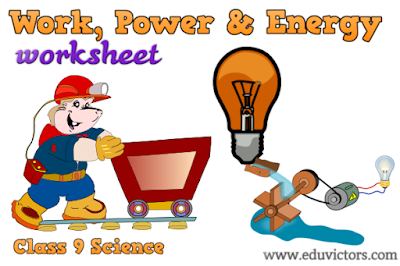## Monday, 31 December 2018

### CBSE Class 9 - Physics - Work, Power and Energy - Worksheet (#eduvictors)(#cbsenotes)

Class 9 - Physics

## Work, Power and Energy

Worksheet

Fill in the blanks with suitable words.

1. Energy is the __________ to do work. Its S.I. unit is ________.

2. Work is force multiplied by ___________ in the direction of force.

3. If the angle between force and the displacement caused is acute then work is said to be ________.

4. If the angle between force and the displacement caused is obtuse, the work is said to be _______.

5. One joule of work is said to be done when a force of ______ displaces a body through a distance of ________ in its own direction.

6. Mechanical energy is of two types i.e., (i) _________ energy (ii) ________ energy

7. Kinetic energy is the energy possessed by a body by virtue of its ________.

8. Potential energy is the energy possessed by a body by virtue of its ________ or __________.

9. According to law of conservation of energy, energy can neither be ________ nor can it be  but it can be _____________ from one body to another.

10. According to the work-energy theorem, the work done by a force on a moving body is equal to
the increase in its ________ energy.

11. Gravitational Potential energy, P = ______

12. 1 kilowatt hour =  J

13. Work has only _______, no _________. So work is a scalar.

14. The sum of the kinetic energy and the potential energy of an object is called its ________ energy.

15. _________ is defined as the rate of doing work or rate of transfer of energy.

1: capacity, joule
2: displacement
3: positive
4: negative
5: 1 newton, 1 metre
6: Kinetic, Potential
7: motion/speed.
8: position, configuration
9: created, destroyed, transferred
10: kinetic
11: mgh
12: 3.6 × 10⁶J
13: magnitude, direction
14: mechanical
15: Power